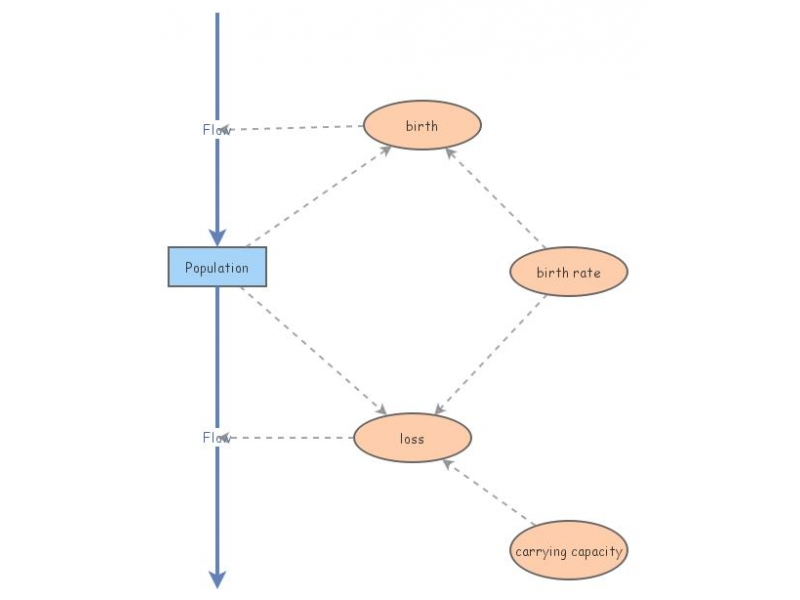Clone of Logistic GrowthThis simple model demonstrates logistic growth.The differential equation looks like

y'(t)=by(t)(1-y(t)/K)

where K is the carrying capacity of the quantity y.

y'(t)=by(t) - b/K*y(t)^2

so the loss term is of the form b/K.

A comparable Mathematica file is available at
http://www.nku.edu/~longa/classes/2018spring/mat375/mathematica/LogisticGrowth-and-DecayModel.nb